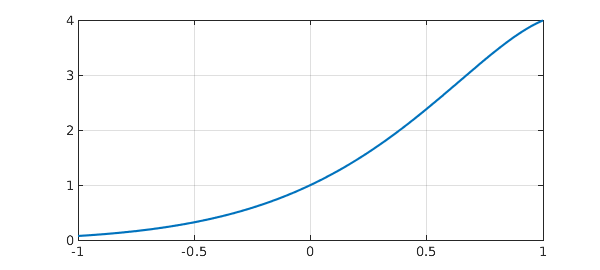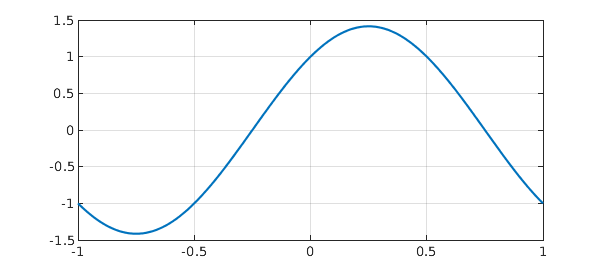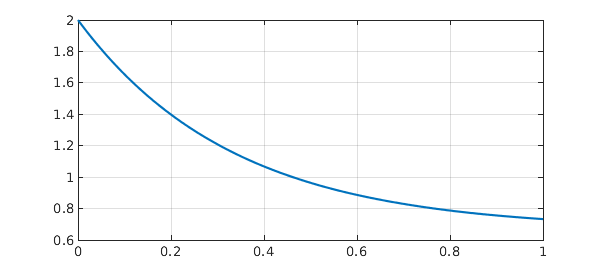Here, we solve three simple linear problems considered in the Wikipedia article on ODEs . The problems are solved in the order they appear in the article, with boundary conditions imposed to make the solutions unique.

### Problem 1: Second-order problem

$$L(y) = y'' - 4y' + 5y = 0, \quad y(-1) = e^{-2} \cos(-1) , ~~ y(1) = e^2\cos(1).$$

Begin by defining the domain $d$, chebfun variable $x$ and operator $N$.

d = [-1 1];
x = chebfun('x',d);
N = chebop(d);

The problem has Dirichlet boundary conditions.

N.lbc = exp(-2)*cos(-1);
N.rbc = exp(2)*cos(1);

Define the linear operator.

N.op = @(y) diff(y,2) - 4*diff(y,1) + 5*y;

Define the right-hand side of the ODE.

rhs = 0;

Solve the ODE using backslash.

y = N\rhs;

Analytic solution.

y_exact = exp(2*x).*cos(x);

How close is the computed solution to the true solution?

norm(y-y_exact)
ans =
3.572143776145396e-14


Plot the computed solution.

plot(y,'linewidth',2), grid on### Problem 2: Simple harmonic oscillator

$$L(y) = y'' + \pi^2 y = 0, \qquad y(-1) = -1, ~~ y'(1) = -\pi.$$

d = [-1 1];
x = chebfun('x',d);
N = chebop(d);
N.op = @(y) diff(y,2) + pi^2*y;

This problem has a Dirichlet boundary condition on the left,

N.lbc = -1;

and a Neumann condition on the right.

N.rbc = @(u) diff(u) + pi;

Define the right-hand side of the ODE.

rhs = 0;

Solve the ODE using backslash.

y = N\rhs;

Analytic solution.

y_exact = cos(pi*x)+sin(pi*x);

How close is the computed solution to the true solution?

norm(y-y_exact)
ans =
2.300835291877525e-14


Plot the computed solution.

plot(y,'linewidth',2), grid on### Problem 3: First-order problem

$$L(y) = y' + 3y = 2 \qquad y(0) = 2 .$$

d = [0 1];
x = chebfun('x',d);
N = chebop(d);

First-order problems require only one boundary condition.

N.lbc = 2;

Define the linear operator.

N.op = @(y) diff(y) + 3*y - 2;

Define the right-hand side of the ODE.

rhs = 0;

Solve the ODE using backslash.

y = N\rhs;

Analytic solution, usually found with integrating factors.

y_exact = 2/3 + 4/3*exp(-3*x);

How close is the computed solution to the true solution?

norm(y-y_exact)
ans =
0


Plot the computed solution

plot(y,'linewidth',2), grid on